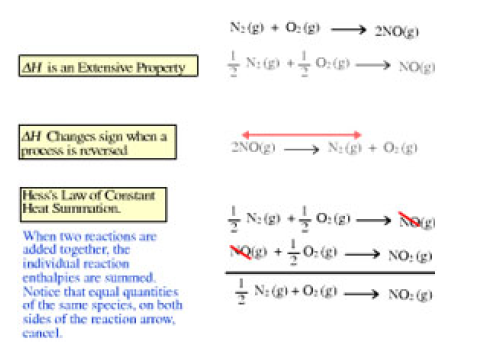# Problem: Click the ΔH is an extensive property button within the activity, and analyze the relationship between the two reactions that are displayed. The reaction that was on the screen when you started and its derivative demonstrate that the change in enthalpy for a reaction, ΔH, is an extensive property. Using this property, calculate the change in enthalpy for Reaction 2.Reaction 1: C3H8(g) + 5 O2(g) → 3 CO2(g) + 4 H2O(g), ΔH1 = − 2043 kJReaction 2: 3C3H8(g) + 15 O2(g) → 9 CO2(g) + 12 H2O(g), ΔH2 = ?Express your answer to four significant figures and include the appropriate units.Open the activity depicting Hess's Law.

###### FREE Expert Solution
97% (167 ratings)View Complete Written Solution
###### Problem Details

Click the ΔH is an extensive property button within the activity, and analyze the relationship between the two reactions that are displayed. The reaction that was on the screen when you started and its derivative demonstrate that the change in enthalpy for a reaction, ΔH, is an extensive property. Using this property, calculate the change in enthalpy for Reaction 2.

Reaction 1: C3H8(g) + 5 O2(g) → 3 CO2(g) + 4 H2O(g), ΔH= − 2043 kJ
Reaction 2: 3C3H8(g) + 15 O2(g) → 9 CO2(g) + 12 H2O(g), ΔH2 = ?

Express your answer to four significant figures and include the appropriate units.

Open the activity depicting Hess's Law.